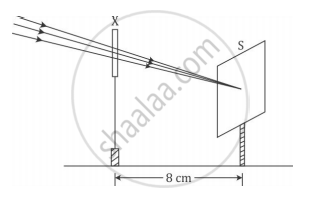# A student used a device (X) to obtain/focus the image of a well illuminated distant building on a screen (S) as shown below in the diagram. Select the correct statement about the device (X). - Science

MCQ

A student used a device (X) to obtain/focus the image of a well illuminated distant building on a screen (S) as shown below in the diagram. Select the correct statement about the device (X).#### Options

• This device is a concave lens of focal length 8 cm.

• This device is a convex mirror of focal length 8 cm.

• This device is a convex lens of focal length 4 cm.

• This device is a convex lens of focal length 8 cm.

#### Solution

This device is a convex lens of focal length 8 cm.

Explanation:

The incident rays after passing through the lens converge at the focus. So, the device 'X' is a converging or a convex lens. The distance between the lens and the screen gives the focal length of the lens.

Concept: Spherical Mirrors
Is there an error in this question or solution?
2014-2015 (March) All India Set 1

Share